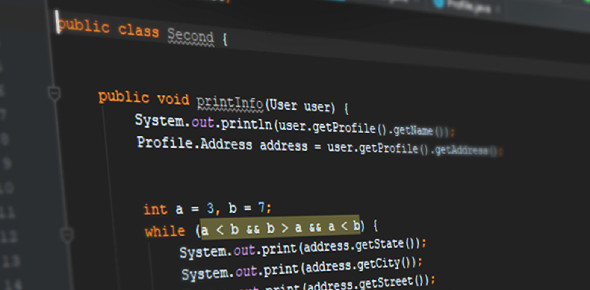# Java Programming MCQ Exam: Quiz

10 Questions | Total Attempts: 430SettingsCreate your own Quiz.

• 1.
Int i; int sum=0; for(i=2;i<=10;i+=2)    sum+=i; System.out.println(""+sum); what is the output of the above program?
• A.

28

• B.

30

• C.

32

• D.

34

• 2.
String str1="Raj",str2="Kumar"; String str3=str1+ " " + str2; System.out.println(""+str3); what  is the output of the above program?
• A.

RajKumar

• B.

Raj Kumar

• C.

Rajeev Kumar

• D.

Null

• 3.
Int X=10; int a=12,b=5; int c=(int)a/b; switch(c) {    case 1:              X=X+2;    case 2:             X=X + 2;     default :            X=X+1; } System.out.println(""+X);
• A.

15

• B.

13

• C.

10

• D.

12

• 4.
Int day=5; switch(day) {   case 10:                System.out.println("India");   default :              System.out.println("Invalid"); } What will be the output of the above program?
• A.

India

• B.

Invalid

• C.

Error

• D.

No output

• 5.
In which phase you will check if the software is according to user specifications?
• A.

Maintenance

• B.

Deployment

• C.

Testing

• D.

Coding

• 6.
What will happen if you do not specify a condition in delete statement?
• A.

No records will be deleted

• B.

All records will be deleted

• C.

Some records are deleted

• D.

Some records are not deleted

• 7.
Which of the following are open source?
• A.

Microsoft Office

• B.

Netbeans

• C.

MYSQL

• D.

Outlook Express

• 8.
Select length('Raj Kumar');
• A.

8

• B.

9

• C.

10

• D.

11

• 9.
Net beans is an IDE?
• A.

True

• B.

False

• 10.
Which of the following are DML statements?
• A.

Insert

• B.

Update

• C.

Delete

• D.

Create

• E.

Drop

Related TopicsBack to top
×

Wait!
Here's an interesting quiz for you.